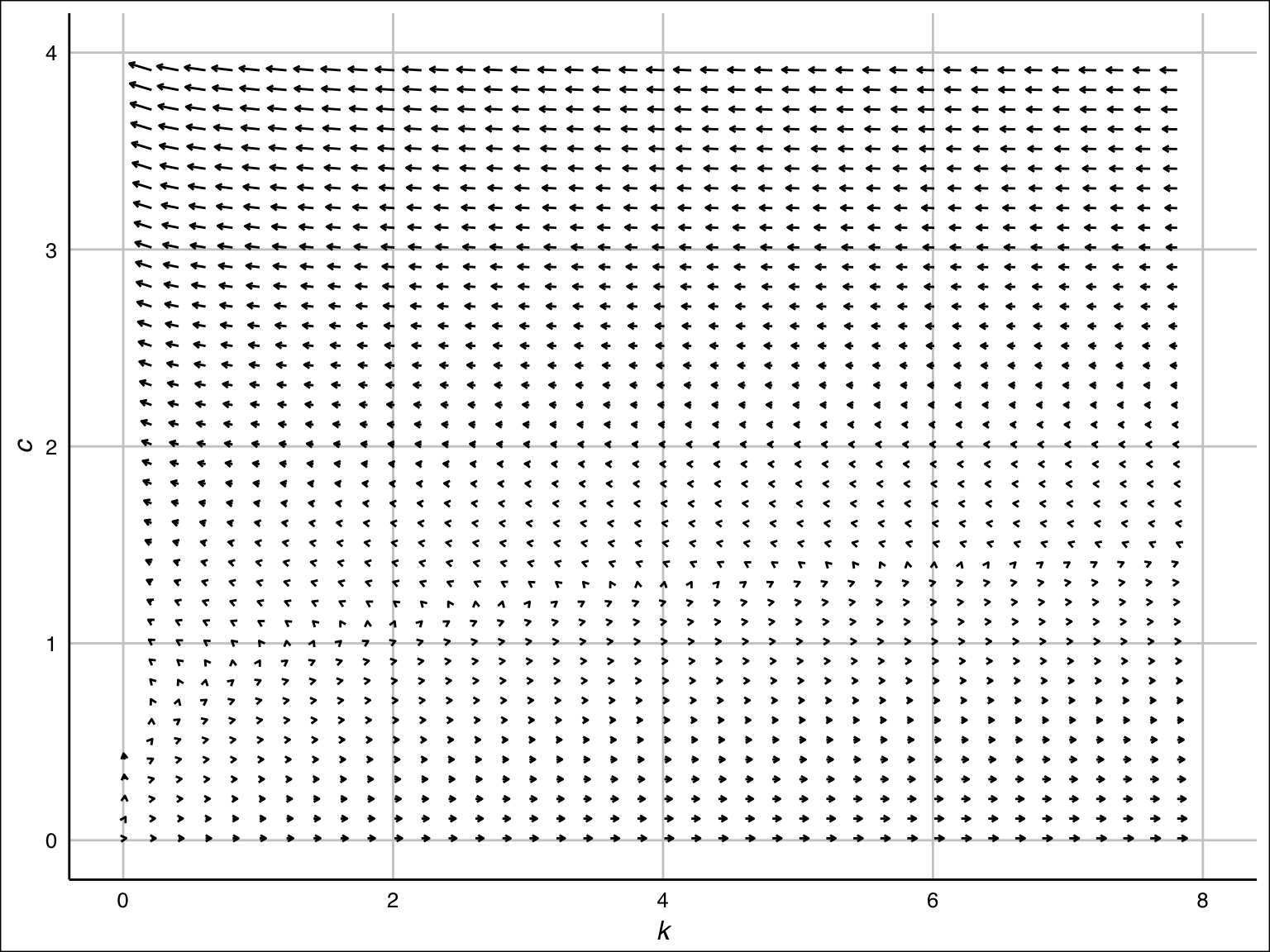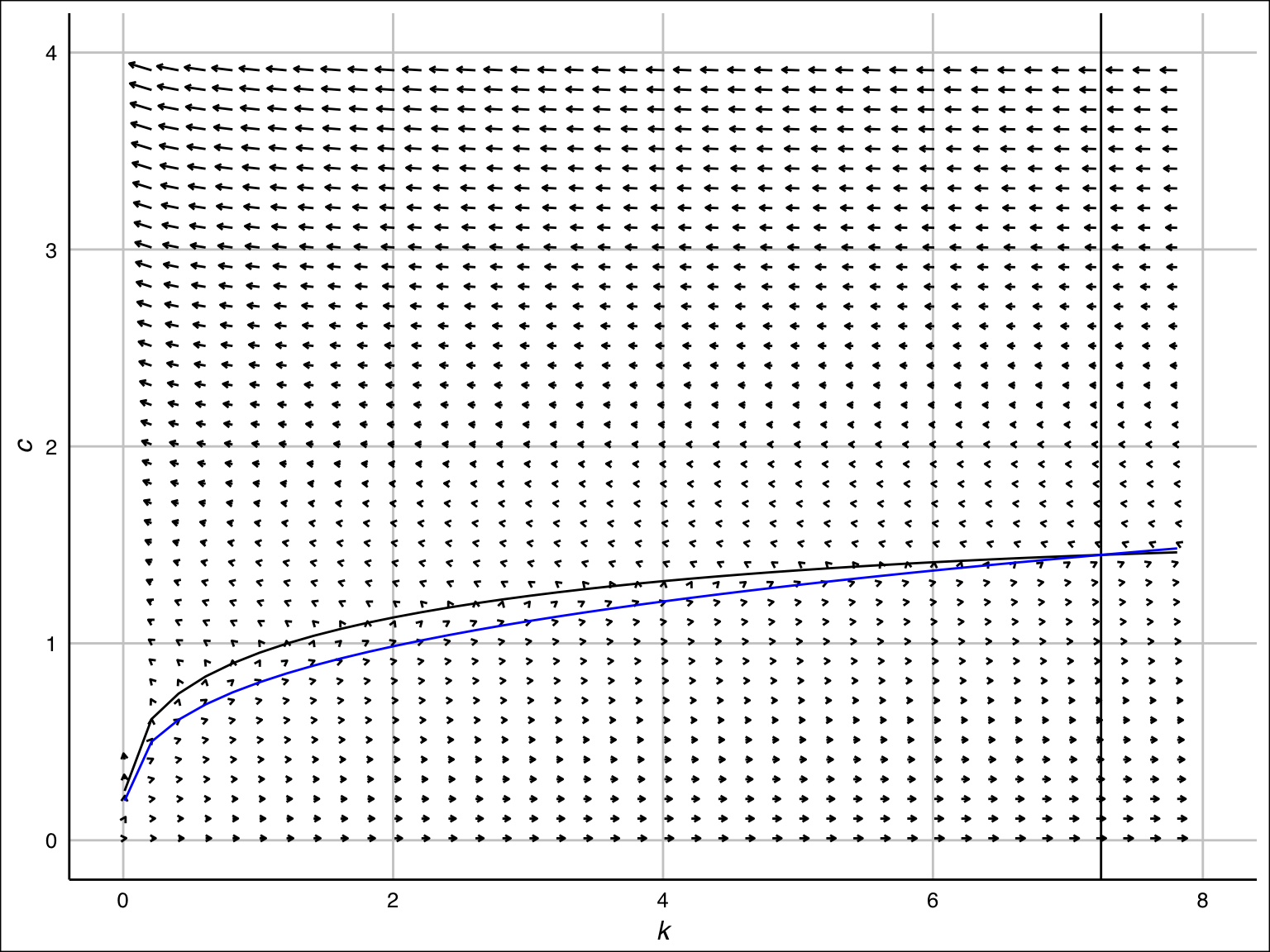January 11, 2017

# Optimal Growth

We have a formulation of the optimal growth problem as follows:

\begin{aligned} & \max \int_0^\infty e^{-\rho t} u(c(t)) dt \\ &\text{subject to } \\ &\qquad c(t) = f(k(t)) - \delta k(t) - \dot k(t) \ge 0, \\ &\qquad k(t) \ge 0, \\ &\qquad k(0): \text{given}. \end{aligned}

# Trade-off between consumption and investment

Reshape the resource constraint into $c(t) + \dot k(t) = f(k(t)) - \delta k(t).$

It is possible to increase the amount of consumption, $$c(t)$$, at any point in time by reducing investment, $$\dot k(t)$$, at the same moment. Since $$\dot k(t) < 0$$ is allowable (disinvestment), $$c(t)$$ can be arbitrarily large.

# Trade-off between consumption and investment (cont’d)

If $$\dot k(t)$$ is too small, however, the amount of capital will be reduced. This results in the reduced consumption in the future because the representative agent cannot continue $$\dot k(t) < - \epsilon < 0$$ forever because $$k(t) \ge 0$$ must hold.

The optimal growth model (and the Ramsey model) is a problem of intertemporal resource allocation.

You need to sacrifice future consumption to consume today.

# Solving the optimal growth problem

We study the solution method next week. Today, we make clear what we mean by solution and draw a sketch of our journey.

The optimal growth model is a problem to find a function $$c(\cdot)$$ or equivalently $$\dot k(\cdot)$$ (Why equivalent?) that maximizes the total utility.

For the representative agent to make a viable decision, the optimal $$c(t)$$ must not depend on the future information; i.e., $$c(t)$$ is a function of $$\{(c(\tau) \mid \tau < t\}$$ and $$\{ k(\tau) \mid \tau \le t \}$$

# Solving the optimal growth problem (cont’d)

We included $$k(t)$$ in the information set. This is because at time $$t$$, $$k(t)$$ should be known in a similar way like $$k(0)$$ is known at $$t = 0$$.

(At time $$t$$, we know the beginning-of-period capital $$k(t)$$ of period $$[t, t+\Delta t)$$. In contrast, we must determine the amount of consumption $$c(t)\Delta t$$ that will happen in the same period.)

# Predetermined/Non-predetermined

Jargons you should learn: - Variables like $$k$$ are called predetermined or state variables. - Variables like $$c$$ are called non-predetermined or control variables.

# What solution means

To solve a model is to write down non-predetermined variables by

• current and past (from the perspective of $$t$$) values of predetermined variables
• current and past values of exogenous variables, and
• fundamental parameters ($$\rho$$, $$\delta$$, $$f$$, $$u$$).

FYI: Modern macroeconomic models may include expectation terms to the list (and eliminate them by restricting shock components to VAR; See the beautiful paper of Blanchard and Khan 1980 in Econometrica and their successors such as Klein 2000 in Journal of Economic Dynamics and Control).

# Differential equations governing dynamics

The first step to determine the dynamics of a model is to obtain the differential equation that the state and control variables obey: i.e., to obtain something like

\begin{aligned} \dot k (t) &= ... \\ \dot c (t) &= ... \end{aligned}

In our particular case, the …’s only contain the current ($$t$$) value of the predetermined and nonpredetermined variables and fundamentals.

# Differential equations governing dynamics (cont’d)

In fact, you already know the dynamics of $$k$$:

\begin{aligned} \dot k (t) &= f(k(t)) - \delta k(t) - c(t) \\ \dot c (t) &= ... \end{aligned}

The dynamics of $$k(\cdot)$$ is determined by the capital accumulation, which is intuitive.

Our effort will be put to derivation of the dynamics of $$c(t)$$.

# Euler Equation

As you will see later, the full dynamics of $$c(\cdot)$$ and $$k(\cdot)$$ is governed by

\begin{aligned} \dot k (t) &= f(k(t)) - \delta k(t) - c(t) \\ \dot c (t) &= \frac{u'(c(t))[f'(k(t)) - \delta - \rho]}{- u''(c(t))} = \frac{u'(c(t))(r(t) - \rho)}{- u''(c(t))}, \end{aligned}

where $$r(t) = f'(k(t)) - \delta$$ is net rate of return on capital.

When the rental rate (instantaneous reward of owning capital) is greater than the discount rate (instantaneous cost of not consuming), the representative agent decides to spend this profits to increase consumption somewhat.

# Vector Field# Problem solved?

At this stage, the problem is not solved yet. Because we have two dimensional dynamical system with only one initial condition $$k(0)$$.

We need one more!

As it will turn out, the dynamics is fully determined by the terminal condition called transversality condition, which states that the representative agent will use up all his capital in the infinite future.

If he died with capital left unconsumed, he would fail to maximize utility.

# Transversality condition

It is known that, under a mild set of conditions (See Kamihigashi 2001, Econometrica), transversality condition (not die before the present value of capital is zero)

$\lim_{t\to\infty}-e^{-\rho t}u'(c(t))k(t)=0$

is necessary for optimality; i.e., among infinitely many paths that satisfy the above mentioned system of differential equations, those that satisfy the transversality condition are optimal.

In our case, we can specify only one solution.

# Simple Example

Let’s see what the Transversality Condition means by a simple example.

\begin{aligned} &\max\left[\ln c_{0}+\beta\ln c_{1}\right]\\ &\text{subject to}\\ &\qquad c_{0}=k_{0}^\alpha - k_{1},\\ &\qquad c_{1}=k_{1}^\alpha - k_{2},\\ &\qquad k_{0}:\text{ given}. \end{aligned}

# Lagrangian

If $$k_2$$ had positive amount at the optimum, you could increase $$c_2$$ by reducing $$k_2$$; since this manipulation results in higher utility, $$k_2 > 0$$ never happens in optimum.

I hope that some of you tried to solve this problem with the following Lagrangian and that you didn’t make it.

$\mathcal L = \ln c_0 + \beta \ln c_1 + \lambda_0 (k_0^\alpha - k_1 - c_0) + \lambda_1 (k_1^\alpha - k_2 - c_1)$

# Imputed Price

$\lim_{t\to\infty}-e^{-\rho t}u'(c(t))k(t)=0$

Price of capital = Cost of increasing one unit of capital = utility cost of reducing consumption. Recall that (relative) price of a good in the perfectly competitive market is determined by the (relative magnitude of) marginal utility. Although there is no market in the optimal growth model, $$u'(c(t))$$ may be thought of as the price (imputed price).

If measured in the unit of utility, it’s $$u'(c(t))$$. $$u'(c(t)) k(t)$$ is the current value of capital measured in the unit of utility. $$e^{-\rho t}u'(c(t))$$ is the present price of period-$$t$$ capital.

$$e^{-\rho t}u'(c(t)) k(t)$$ is the present value of period-$$t$$ capital. The present value of positive amount of capital in the infinite future should be zero. If it’s positive, $$u'(c(t))k(t)$$ grows indefinitely and the representative agent cannot maximize his utility.

A candidate of the solution is the path that converges to a constant; i.e.,

$(k(t), c(t)) \to (k^*, c^*)$ Since $$e^{-\rho t} \to 0$$ and $$u'(c(t))k(t) \to u'(c^*) k^*$$, we have the transversality condition.

\begin{aligned} \dot k (t) &= f(k(t)) - \delta k(t) - c(t) \\ \dot c (t) &= \frac{u'(c(t))[f'(k(t)) - \delta - \rho]}{- u''(c(t))} = \frac{u'(c(t))(r(t) - \rho)}{- u''(c(t))}, \end{aligned}

From the dynamic equations, we can easily find the steady state $$(k^*, c^*)$$, which solve the following system of equations:

\begin{aligned} c &= f(k) - \delta k \\ k &= (f')^{-1} (\delta + \rho). \end{aligned}

Exercise: Show that the steady state $$(k^*, c^*)$$ satisfies the above system of equations. [Hint: What was the definition of steady state?]

# Optimal Path

In the optimum, the economy moves along the blue curve toward the steady state. By finding such curve we obtain the optimal consumption function ($$k(t) \mapsto c(t)$$), which maps the predetermined variable to non-predetermined variable.# Quantitative analysis with CRRA utility

Working with CRRA utility function is useful.

$u(c) = \left\{ \begin{array}{ll} \frac{c^{1-\theta} - 1}{1 - \theta}, & \theta \ge 0,\ \theta \neq 1, \\ \ln c, & \theta = 1. \end{array} \right.$

With this utility function, the dynamic system can be written as

\begin{aligned} \dot k (t) &= f(k(t)) - \delta k(t) - c(t) \\ \dot c (t) &= \frac{c(t)(f'(k(t)) - \delta - \rho)}{\theta} \end{aligned}

# $$\dot k \gtreqqless 0$$

\begin{aligned} \dot k > 0 &\Leftrightarrow f(k) - \delta k - c > 0 \\ &\Leftrightarrow c < f(k) - \delta k \end{aligned}

\begin{aligned} \dot k < 0 &\Leftrightarrow f(k) - \delta k - c < 0 \\ &\Leftrightarrow c > f(k) - \delta k \end{aligned}